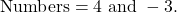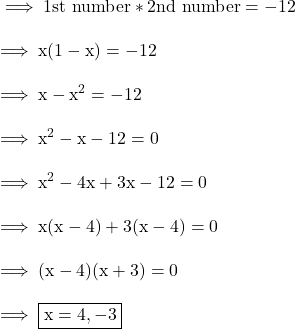## Find the TWO integers whos product is -12 and whose sum is 1 ​

Question

Find the TWO integers whos product is -12 and whose sum is 1

in progress 0
1 month 2021-08-02T10:27:21+00:00 1 Answers 4 views 0Step-by-step explanation:

Given :-

The sum of two numbers is 1 .

The product of the nos . is 12 .

And we need to find out the numbers. So let us take ,

First number be x

Second number be 1-x .

According to first condition :-Hence the numbers are 4 and -3